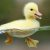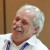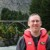# Using Standard Deviation to Set Nominal Target

Six Sigma – iSixSigma Forums General Forums General Using Standard Deviation to Set Nominal Target

Viewing 3 posts - 1 through 3 (of 3 total)
• Author
Posts
• #241175Ahiru-san
Participant

How can I use standard deviation to target nominal?

Say my nominal width dimension is 90 mm.

My Lower Spec Limit is 80 mm and my Upper Spec Limit is 100 mm.

My standard deviation is 2 mm.

So, my Lower Control Limit is 84 mm while my Upper Control Limit is 96 mm (that is 3 standard deviations either way). I have good process capability, but it with that much variation (+/- 6 mm), how do I target nominal?

Please check my logic here.

A. Zero Standard Deviations = If I say we cannot start until I measure a nominal width of 90, I know that I will have a lot of setup time because the process normally varies from 84-96. This is normal variation. So that won’t work.

B. 1 Standard Deviation = If I start anywhere from 88 to 92. 88-6= 82 and that is inside my LSL. 92+6=98 and that is inside my USL. So, nominal +/- 1 standard deviation will work, but may be require additional setup time.

C. 2 Standard Deviations = I can start anywhere from 86 to 94 that means 86-6=80 and that just reaches my LSL. While 94+6=100 and that also is technically in spec. So, that would work, but just barely.

D. 3 Standard Deviations = If I use my control limits of 84-96, I know the process may vary normally from a low of 84-6=78 (which is below my tolerance of 80), and it could go as high as 96+6=102 (which is above my USL of 100). Okay, so that won’t work.

Is this a reasonable approach?

Is there some standard way of doing this?

Have I missed something?

0
#241181Mike Carnell
Participant

@Ahiru-san Where inside the spec is the most profitable place for your company to produce the product? You might want to understand the Taguchi Loss Function.

1
#241198Chris Seider
Participant

Interesting query.

Have you put together a mathematical model to help answer your questions and @mike-carnell has given you a hint to an approach.

keep in mind you’re making lots of assumptions…would you be using an Xbar chart–if so I’d doubt your CL’s would be as you’ve concluded.

1
Viewing 3 posts - 1 through 3 (of 3 total)

You must be logged in to reply to this topic.